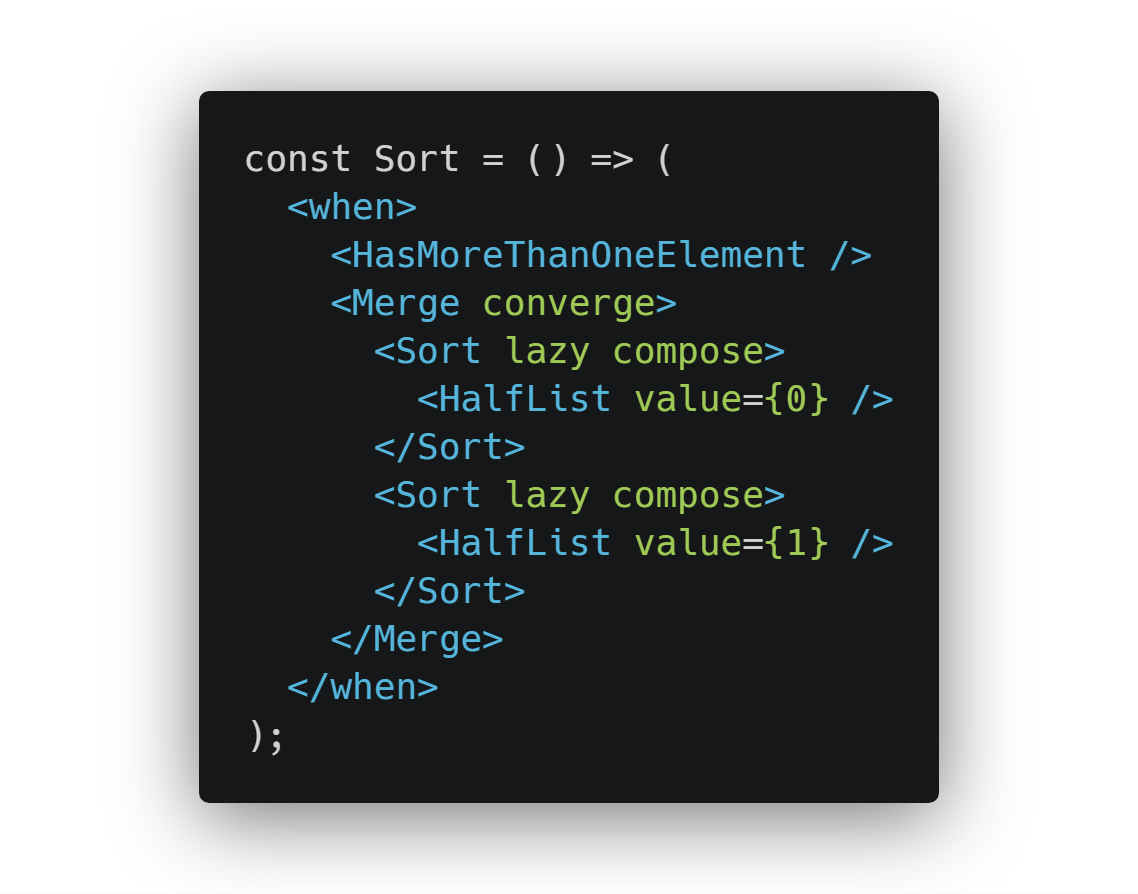QAQMiao

## JSX 这么6？#### JSX

``````function getGreeting(name) {
return (
<div className="greeting">
<h1 foo="bar">Hello!</h1>
<h2>Good to see you {name}</h2>
</div>
);
}

``````

``````function getGreeting(name) {
return React.createElement(
"div",
{ className: "greeting" },
React.createElement("h1", { foo: "bar" }, "Hello!"),
React.createElement("h2", null, "Good to see you ", name)
);
}

``````

Babel REPL

`React.createElement`是 React 用来创建元素的方法。因为JSX通常与React同时使用，所以 Babel 默认注入这个方法，但实际情况并非如此。事实上，JSX 是从React中脱离出来的，JSX 是用于在 JS 中使用类似 XML 的语法定义树结构的规范。这个树结构可以是一个 React 组件渲染的元素，也可以是完全不同的其他的东西。

``````/** @jsx foo */
var bar = <x>Hi</x>;
var bax = <Y>Hi</Y>;

// becomes:
var bar = foo("x", null, "Hi");
var bax = foo(Y, null, "Hi");

``````

#### 数学中的JSX

``````const Sum = (...args) => args.reduce((a, b) => a + b, 0);
const Pow = ({ exponent }, base) => Math.pow(base, exponent);
const Sqrt = x => Math.sqrt(x);

const Hypotenuse = ({ a, b }) => (
<Sqrt>
<Sum>
<Pow exponent={2}>{a}</Pow>
<Pow exponent={2}>{b}</Pow>
</Sum>
</Sqrt>
);

/** @jsx calc */
function calc(operation, props, ...args) {
let params = props ? [props] : [];
params = params.concat(...args);
return operation(...params);
}

console.log(<Hypotenuse a={3} b={4} />);

``````

codepen

``````const Hypotenuse = ({ values }) => (
<Sqrt>
<Sum>{values.map(v => <Pow exponent={2}>{v}</Pow>)}</Sum>
</Sqrt>
);

console.log(<Hypotenuse values={[3, 4, 5]} />);

``````

codepen

#### 无所不能的JSX

``````import R from "ramda";
var divisibleBy = R.curry(R.pipe(R.flip(R.modulo), R.equals(0)));
var fizzbuzz = R.map(
R.cond([
[R.both(divisibleBy(3), divisibleBy(5)), R.always("FizzBuzz")],
[divisibleBy(3), R.always("Fizz")],
[divisibleBy(5), R.always("Buzz")],
[R.T, R.identity]
])
);
console.log(fizzbuzz(R.range(1, 16)));

``````

Code from stackexchange codereview

``````/** @jsx run */
function run(f, props, ...args) {
return R[f](...args);
}

var divisibleBy = (
<curry>
<pipe>
<flip>
<modulo />
</flip>
<equals>{0}</equals>
</pipe>
</curry>
);

var fizzbuzz = (
<map>
<cond>
{[
[
<both>
{divisibleBy(3)}
{divisibleBy(5)}
</both>,
<always>"FizzBuzz"</always>
],
[divisibleBy(3), <always>"Fizz"</always>],
[divisibleBy(5), <always>"Buzz"</always>],
[R.T, <identity />]
]}
</cond>
</map>
);

console.log(fizzbuzz(R.range(1, 16)));

``````

codepen

``````var FizzBuzz = (
<map>
<cond concat>
<pair>
<both>
<DivisibleBy value={3} />
<DivisibleBy value={5} />
</both>
<always value="FizzBuzz" />
</pair>
<pair>
<DivisibleBy value={3} />
<always value="Fizz" />
</pair>
<pair>
<DivisibleBy value={5} />
<always value="Buzz" />
</pair>
<pair>
<t />
<identity />
</pair>
</cond>
</map>
);

console.log(
<FizzBuzz>
<range>
{1}
{16}
</range>
</FizzBuzz>
);

``````

codepen